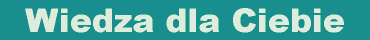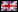wprowadź własne kryteria wyszukiwania książek: (jak szukać?) książki polskie książki zagraniczne polskie i zagraniczne tytuł autor wydawca opis wszędzie
 Twój koszyk:   0 zł   zamówienie wysyłkowe >>>
Strona główna > opis książkiEnglish version
Książki:

Zamów informacje o nowościach z wybranego tematu

Informacje:

Szukasz książki?
Pomożemy Ci!
512 994 090

Napisz
poczta@ksiazki24h.pl# BATÓG B. BIESZK-STOLORZ B. FORYŚ I. GUZOWSKA M. HEBERLEIN K.

wydawnictwo: DIFIN , rok wydania 2021, wydanie I

cena netto: 73.15 Twoja cena  69,49 zł + 5% vat - dodaj do koszyka

## Mathematics for students of economics, finance and management

The textbook Mathematics for Students of Economics, Finance, and Management has been prepared mostly for students in the disciplines: Economics and Finance and Quality and Management Sciences. However, it can also be used by students of other fields of studies with courses related to mathematics, algebra, and mathematical analysis.

The theory is presented in an accessible way necessary to solve the proposed tasks. Each chapter contains examples to help to understand the theory and exercises to be solved by students (with answers). This form of presentation of individual issues facilitates individual work, especially for part-time students.

The textbook has been supplemented with a chapter containing practical usage of mathematics in economics, finance, and management. The textbook is the result of many years of teaching experience of the authors during lectures, exercises and consultations with students. All of the chapters are written by scholars working in the Department of Econometrics and Statis- tics, Institute of Economics and Finance at the University of Szczecin.

Preface

Chapter 1. INITIAL ISSUES

1.1. Logical sentences and their properties
1.2. Propositional function. Quantifiers
1.3. Set algebra
1.4. Cartesian product
1.5. Relations
1.6. Functions
1.7. Operations and groups
1.8. Vector space and its properties

Chapter 2. MATRICES

2.1. Matrix definition and types
2.2. Operations on matrices
2.3. The determinant of a square matrix
2.4. Inverse matrix
2.5. The rank of a matrix
2.6. Matrix equations

Chapter 3. SYSTEMS OF LINEAR EQUATIONS

3.1. Definition and types of systems of linear equations
3.3. Methods of solving systems of linear equations
3.4. Homogeneous systems of linear equations
3.5. Gaussian elimination
3.6. Eigenvalues of matrices

Chapter 4. LIMITS AND CONTINUITY

4.1. Metric space
4.2. Sequences and their properties
4.3. Limits of sequences
4.4. Limits and continuity of functions
4.5. Asymptotes

Chapter 5. DERIVATIVE OF A FUNCTION AND ITS APPLICATIONS

5.1. Derivative and differential of functions
5.2. Monotonicity and extrema of functions
5.3. Concavity and inflection points
5.4. L'Hospital's rule
5.5. Sketching graphs of functions

Chapter 6. FUNCTIONS OF MANY VARIABLES AND THEIR EXTREMA

6.1. Definition of a function of many variables
6.2. Partial derivatives
6.3. Higher-order partial derivatives
6.4. Total differential
6.5. Extrema of functions of many variables
6.6. Constrained (conditional) extrema

Chapter 7. INTEGRAL CALCULUS

7.1. Indefinite integral and its properties
7.2. Integration by substitution
7.3. Integration by parts
7.4. Integration of rational functions
7.5. Definite integral and its properties
7.6. Application of definite integral
7.7. Improper integrals

Chapter 8. APPLICATIONS IN ECONOMICS, FINANCE, AND MANAGEMENT

8.1. Application of systems of linear equations
8.2. Application to input-output analysis in economics – Leontief model
8.3. The market(s) model and national income models
8.4. Elements of financial mathematics
8.5. Application of differential calculus
8.6. Application of integral calculus

Appendix 1. ELEMENTARY FUNCTIONS AND THEIR PROPERTIES

Appendix 2. SPECIAL MATHEMATICAL FORMULAS

Index
References

252 pages, Paperback

Po otrzymaniu zamówienia poinformujemy,
czy wybrany tytuł polskojęzyczny lub anglojęzyczny jest aktualnie na półce księgarni.